﻿ Solution of Poisson’s Equation Using Artificial Neural NetworksComputer Science and Engineering

p-ISSN: 2163-1484    e-ISSN: 2163-1492

2021;  11(1): 17-21

doi:10.5923/j.computer.20211101.03

Received: May 19, 2021; Accepted: May 31, 2021; Published: Jun. 15, 2021### Solution of Poisson’s Equation Using Artificial Neural Networks

Jay P. Narain

Retired, Worked at Lockheed Martin Corporation, Sunnyvale, CA, USA

Correspondence to: Jay P. Narain , Retired, Worked at Lockheed Martin Corporation, Sunnyvale, CA, USA.
 Email: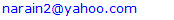Abstract

The solution of Partial differential equations has been of considerable interest lately because of interest in Machine Learning methods. The use of artificial neural network to solve ordinary and partial differential equations has been elaborately described in the works of Lagaris, Likas and Fotiadis . Although finite difference, finite element, and other numerical and analytical methods are computationally more efficient, the machine learning methods like artificial neural network offer the hope of solving complicated non-linear equations without deriving analytical methods. The neural network method  uses boundary conditions embedded with neural network. Some neural network experience is required to use such method. Presently a very simple neural network scheme is presented where boundary conditions are explicitly applied in the loss function. The neural network differentiation method is based on the work of Luis Angel .

Keywords: Differential equations, Ordinary and partial, Neural netwok, Network differentiation, Machine learning methods

Cite this paper: Jay P. Narain , Solution of Poisson’s Equation Using Artificial Neural Networks, Computer Science and Engineering, Vol. 11 No. 1, 2021, pp. 17-21. doi: 10.5923/j.computer.20211101.03.

1. Introduction
2. Discussions
3. Examples
4. Conclusions

### 1. Introduction

The ordinary and partial differential equations have been solved anaytically and numerically for many years. Various numerical schemes were developed with the advent of computers. From such schemes, the finite difference scheme has been a popular numerical method. Findiff library from PyPl offers a Python package to solve such equations in any number of dimensions . The boundary condition based neural network method for non linear Blasius equation using autogad library  was investigated by Kitchin . This work was extended to more complicated Falkar-Skan equations by Narain . The neural network schemes require derivative of neural network. The autograd library  was used in these methods for equations in one dimension. The present work is aimed at solving Poisson’s equation in two dimensions. This equation is used in many engineering fields such as fluid dynamics, electrostatics, traffic control system, etc. Angel  has developed a nice scheme for multi -dimensional neural network and their derivatives. With this scheme, any ordinary and partial differential equation can be solved with ease. Most of the applications have been for Laplace equation which is Poisson’s equation with no source term. Several examples for Poisson’s equation will be presented together with comparison with finite difference scheme.

### 2. Discussions

Poisson’s equation is
𝛥𝛟 = f
where 𝛥 is the Laplace operator, and f and 𝛟 are real or complex valued functions on a manifold. Usually, the source function f is given and 𝛟 is sought. When the manifold is Euclidean space, the Laplace operator is often denoted as 𝛻2 and so Poisson’s equation is frequently written as
𝛻2 𝛟 = f
In two-dimensional cartesian coordinates, it takes the form
d2 𝛟(x,y)/dx2 + d2 𝛟(x,y)/dy2 = f(x,y)
when f =0, we obtain Laplace’s equation. cartesian coordinates.
To solve any equation, boundary conditions are needed. There are three type of boundary conditions (b.c.), for example boundary condition on edge y=0 would be
𝛟(x,0) = a(x)
which is the Dirichlet Boundary condition, and
d 𝛟(x,0)/dn = b(x)
which is Neumann boundary condition. A mixture of Dirichlet and Neumann is termed as mixed boundary condition. dn denoted normal direction on an edge.
There are several ways, this equation can be solved. Exact solution can be obtained using Green's function and other methods. Very fast and accurate numerical solution can be obtained using finite difference, finite volume, finite element and other methods. The matrix inversion methods are efficient for small dimensions, Jacobi and Gauss-Seidel iterative methods are good for any dimension and any boundary condition. The gradient decent and conjugate gradient methods are more efficient but are limited to zero Dirichlet boundary conditions on the edges.
Following ref 2., the neural network for a two-dimensional case is defined as
Define neural_network(x,W) as nnet: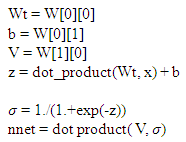The important neural net derivative function is defined as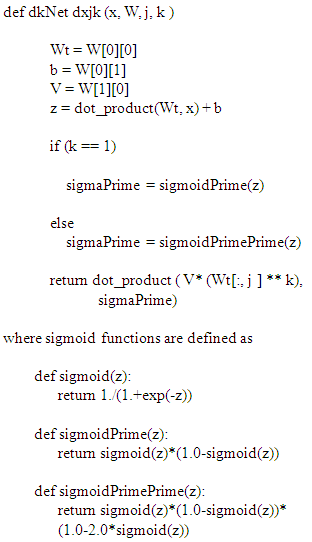Here x is the input vector with two coordinates (x,y), W is the network weight and b is the bias, j denoted direction of partial derivatives (0 for x, 1 for y), and k stands for partial derivative order ((1 for first order, 2 for second order).
The loss function is defined as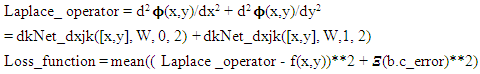Where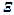is the summation indicator for all the boundary condition errors. An example of b.c error will be 𝛟(x,0) - a(x).
Next the gradient of the loss_sum is minimized by Adam scheme . In general, the nnet function is initialized with random numbers. Thus, the initial solution will look totally different from expected solution. After five hundred iterations, the solution converges. The predicted solution matches pretty well with the FinDiff or exact solution.
Next we will examine two cases from ref. 1. One has Dirichlet boundary condition. Other one can be solved both in Dirichlet b.c. mode or as a mixed b.c. mode. We shall use mixed b.c. mode as it will show the ease of problem setup for such case.
The complete Python program for this paper can be downloaded from narain42/Poission-s-Equation-Solver-Using-ML on github.com.

### 3. Examples

The first example, Problem 7 of ref. 1, is a very simple case with zero Dirichlet b.c. on three edges, and a non-zero either Dirichlet or Neumann b.c. on one edge. The following are the details:
𝛻2 𝛟 (x, y) = (2. – π2y2) sin(πx)
with x, y ϵ [0,1] and with mixed b.c, 𝛟 (0, y) = 0, 𝛟 (1, y) = 0, 𝛟 (x,0) = 0, and d 𝛟(x, 1)/dy = 2 sin(πx).
First the FinDiff , finite-difference solution is obtained. The solution plots are shown below.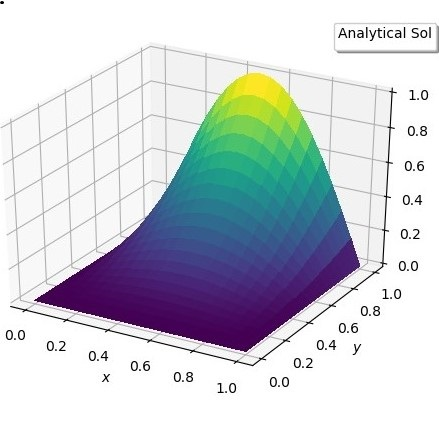Figure 1(a). Exact solution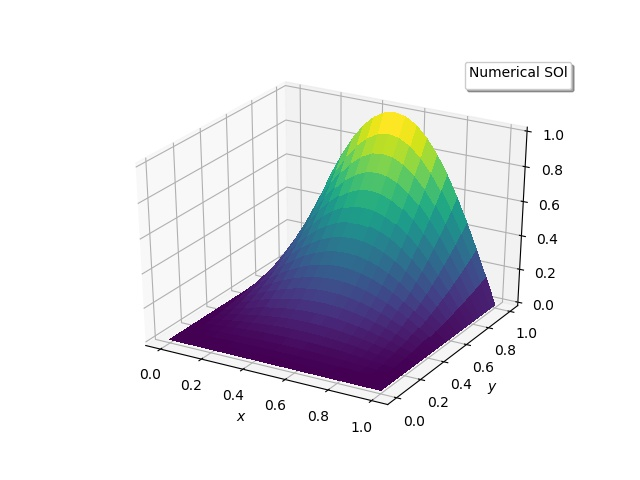Figure 1(b). Numerical Solution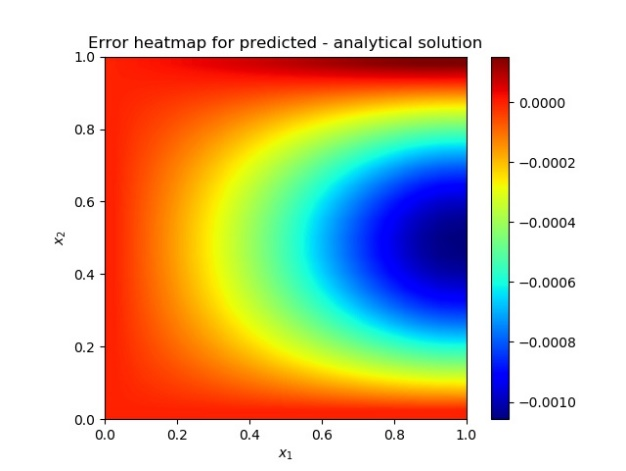Figure 1(c). Error between solutions
For Artificial Neural network solution , the boundary conditions are embedded in the neural network . The suggested trial solution for Neumann b.c is
𝛟trial(x,y) = B(x,y) + x(1-x)y[N(x,y,p) – N(x,1,p) -d(N(x,1,p))/dy}
Where B(x,y) is related to the b.c, and N(x,y,p) is related to the neural network.
In the scope of present neural network model, differentiating the trial function for Laplace operator will be a complicated task. The simple boundary condition scheme model is as follows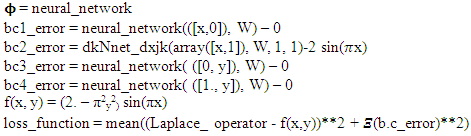This is all what is required to do the Adam  optimization to get the desired solution. The results are: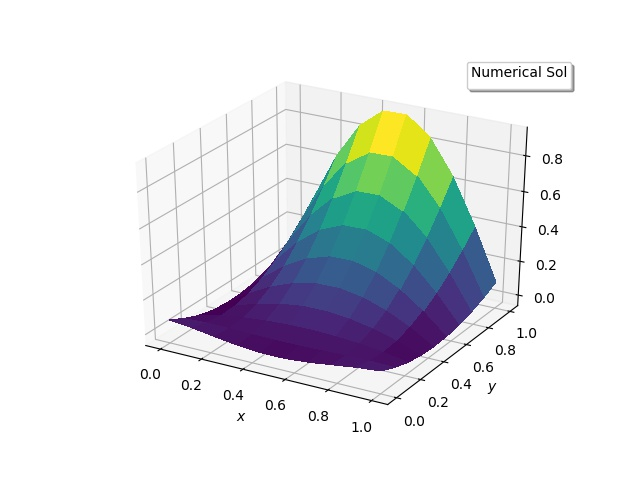Figure 2(a). Numerical solutions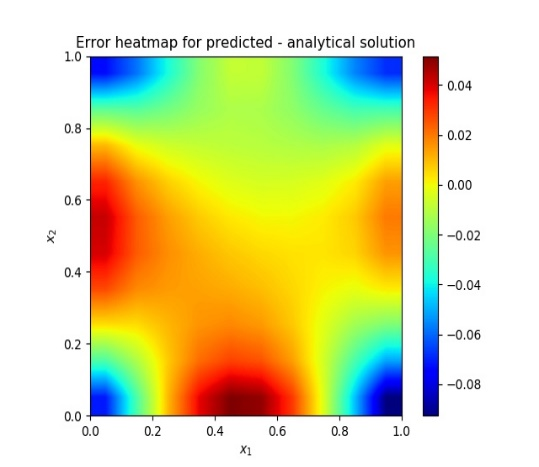Figure 2(b). heatmap of the solution error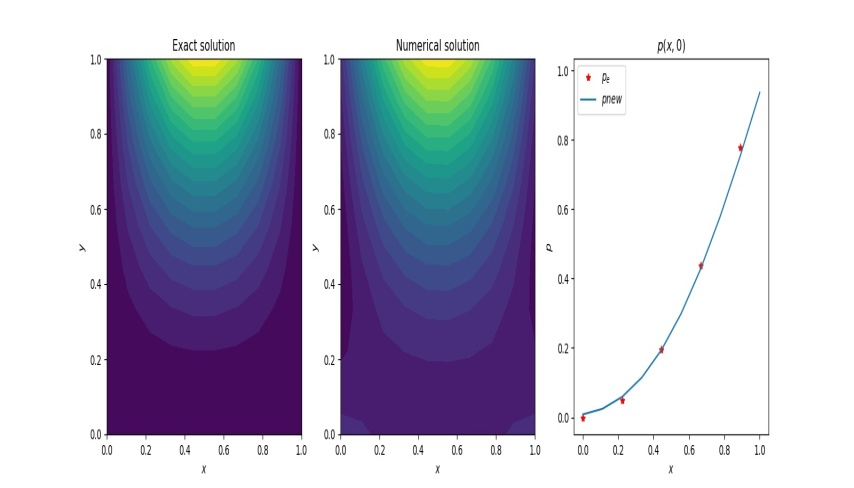Figure 2(c). Contour plots and comparison with exact solution
The FinDiff solver takes less than 2 seconds, while neural network iterations on (21x21) grid domain took around 1760 seconds for 500 iterations.
Next case is problem 5 of ref 1. This has Dirichlet boundary conditions on all edges. The numerical model is as follows: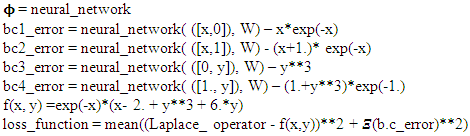This model is used in Adam  optimization to get the desired solution. The Dirichlet b.c. problems converge faster than Neumann conditions. It took only 580 seconds on a (15x15) grid, with 5000 iterations, to converge. No FinDiff results are presented to save the length of this article. The results are similar to those presented below.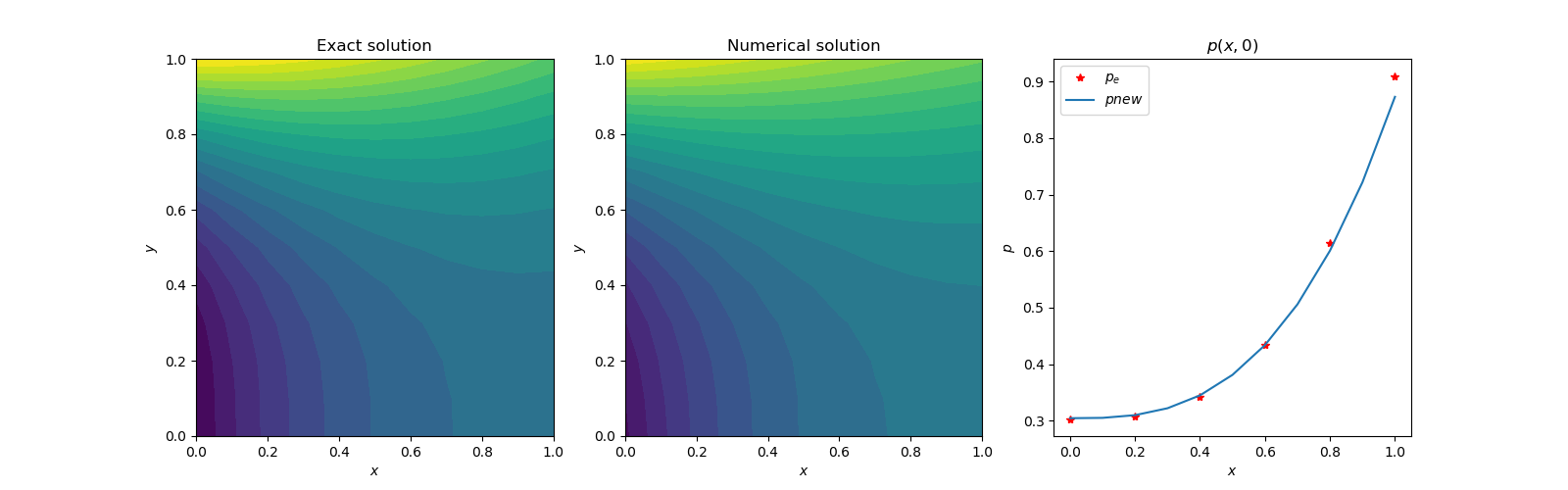Figure 3(a). Contour plots and comparison with exact solution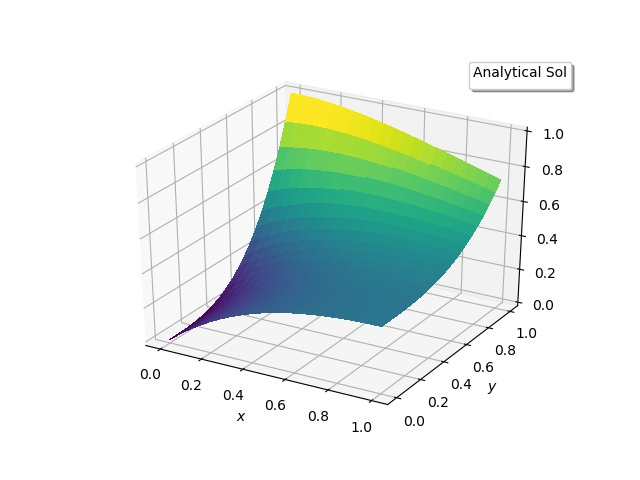Figure 3(b). Exact solution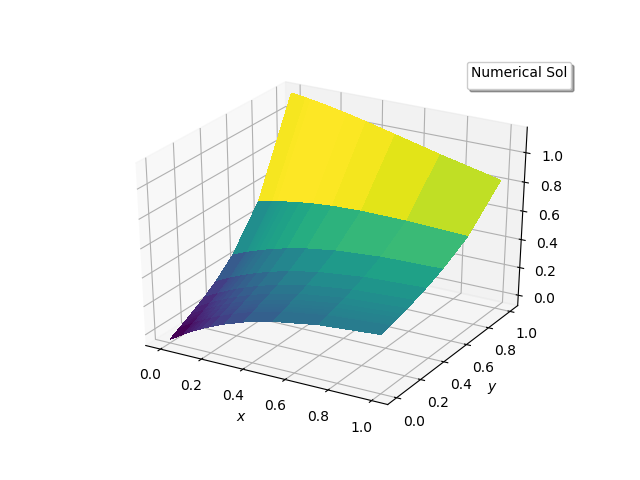Figure 3(c). Numerical solution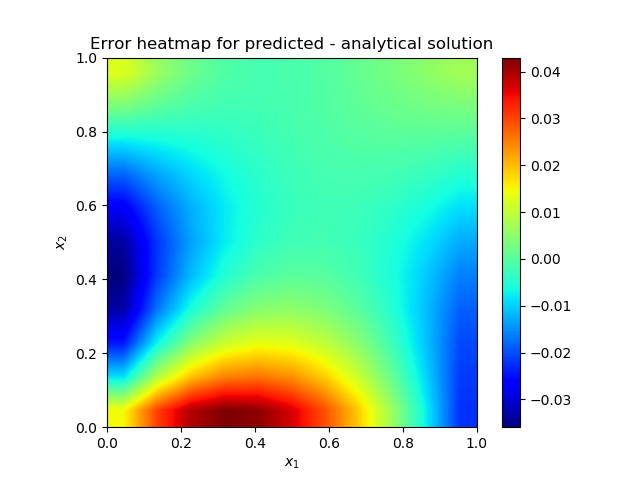Figure 3(d). Heatmap of the solution error

### 4. Conclusions

The new neural network scheme seems to be an efficient way to solve partial differential equations up to second order. The method was applied to first order linear and non-linear partial differential equation cases. Although the results are not reported to keep this paper concise, the results were precisely similar to FinDiff solutions.

### References

  I.E. Lagaris, A. Likas, and D.I. Fotiadis, “Artificial Neural Networks for solving ordinary and partial differential equations,” arXiv: physics/9705023v1, 19th May 1977.  Luis, Angel, “Solving differential equations with neural networks,” imyoungmin/DENN on github.com, May 2, 2019.  Findiff library, pypl.org/project/findiff, Feb 12, 2021.  Ryan Adams, “autograd”, HIPS/autograd on github.com, March 5, 2015.  John Kitchin, “pycse-Python3 Computations in Science and Engineering”, jkitchin@andrew.cmn.edu, 2018.  J.P. Narain, “Exploring Blasius and Falkner-Skan Equations with Python”, American Journal of Fluid Mechanics, Feb 6, 2021.